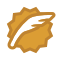# A + B Problem II

Time Limit: 2000/1000 MS (Java/Others)    Memory Limit: 65536/32768 K (Java/Others)
Total Submission(s): 191832    Accepted Submission(s): 36673

Problem Description
I have a very simple problem for you. Given two integers A and B, your job is to calculate the Sum of A + B.

Input
The first line of the input contains an integer T(1<=T<=20) which means the number of test cases. Then T lines follow, each line consists of two positive integers, A and B. Notice that the integers are very large, that means you should not process them by using 32-bit integer. You may assume the length of each integer will not exceed 1000.

Output
For each test case, you should output two lines. The first line is "Case #:", # means the number of the test case. The second line is the an equation "A + B = Sum", Sum means the result of A + B. Note there are some spaces int the equation. Output a blank line between two test cases.

Sample Input
2 1 2 112233445566778899 998877665544332211

Sample Output
Case 1: 1 + 2 = 3 Case 2: 112233445566778899 + 998877665544332211 = 1111111111111111110

import java.math.BigDecimal;
import java.io.BufferedInputStream;
import java.util.Scanner;
public class Main {

/**
* @param args
*/
public static void main(String[] args) {
// TODO Auto-generated method stub
Scanner in = new Scanner(new BufferedInputStream(System.in));
int n, num = 0;
n = in.nextInt();
while(n>=1)
{
n--;
num++;
String a = in.next();
String b = in.next();
BigDecimal big1 = new BigDecimal(a);
BigDecimal big2 = new BigDecimal(b);
BigDecimal big3;
System.out.println("Case "+num+":");
System.out.println(a + " + " + b +" = " + big3.toString());
if(n!=0) System.out.println("");
}
}

}


wangxiaomingCSDN认证博客专家 架构 Spring Boot Redis

08-18486

#### hdu 1002 大数问题02-02176

#### hdu1002 大数相加

01-211727

#### HDU 1002 大数加法（C语言）

12-03572

#### 杭电HDU1002（大数加法）

10-02837

#### HDU1002大数相加问题

12-1433

#### hdu1002 大数运算

07-09750

#### HDU 1002大数加法

05-22653

#### hdu 1002大数相加

03-11304

#### hdu 1002大数加法©️2020 CSDN 皮肤主题: Age of Ai 设计师: meimeiellie点击重新获取扫码支付1.余额是钱包充值的虚拟货币，按照1:1的比例进行支付金额的抵扣。
2.余额无法直接购买下载，可以购买VIP、C币套餐、付费专栏及课程。余额充值